##### Geometry For DummiesOf the three places an angle’s vertex can be in relation to a circle (inside, on, or outside the circle), the two types of angles that have their vertex on a circle — inscribed angles and tangent-chord angles — are the ones that come up in the most problems and are therefore the most important.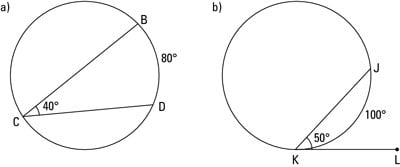• Inscribed angle: An inscribed angle, like angle BCD in the above figure on the left, is an angle whose vertex lies on a circle and whose sides are two chords of the circle.

• Tangent-chord angle: A tangent-chord angle, like angle JKL in the above figure on the right, is an angle whose vertex lies on a circle and whose sides are a tangent and a chord of the circle.

Measure of an angle on a circle: The measure of an inscribed angle or a tangent-chord angle is one-half the measure of its intercepted arc.

For example, in the above figure,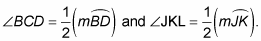Make sure you remember the simple idea that an angle on a circle is half the measure of the arc it intercepts (or if you look at it the other way around, the arc measure is double the angle). If you forget which is half of which, try this: Draw a quick sketch of a circle with a 90° arc (a quarter of the circle) and an inscribed angle that intercepts the 90° arc. You’ll see right away that the angle is less than 90°, telling you that the angle is the thing that’s half of the arc, not vice versa.

Congruent angles on a circle:

• If two inscribed or tangent-chord angles intercept the same arc, then they’re congruent (see the below figure on the left).

• If two inscribed or tangent-chord angles intercept congruent arcs, then they’re congruent (see the below figure on the right).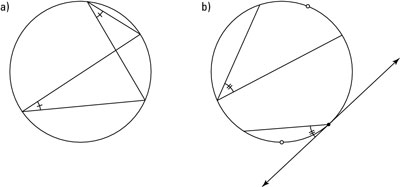Time to see these ideas in action.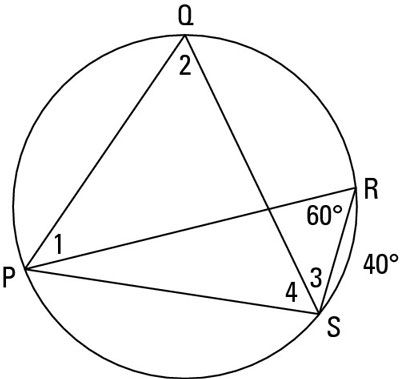Using the figure above, solve the following problem: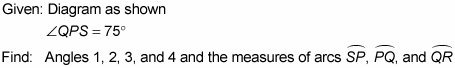The key to this problem is to just use the inscribed angle formula over and over. Remember — the angle is half the arc; the arc is twice the angle.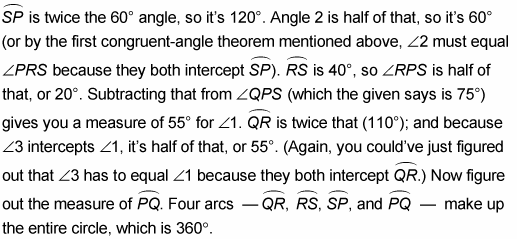You’ve got the measures of the first three: 110°, 40°, and 120° respectively. That adds up to 270°.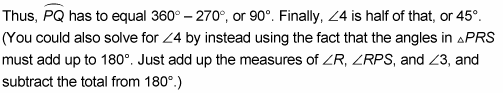Note: This triangle idea also gives you a good way to check your results — do the angles add up to 180°?That does add up to 180°, so it checks, which leads to the following tip.

Whenever possible, check your answers with a method that’s different from your original solution method. This is a much more effective check of your results than simply going through your work a second time looking for mistakes.